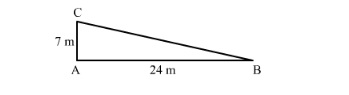# A man goes 24 m due west and then 7 m due north. How far is he from the starting point?`
Question:

A man goes 24 m due west and then 7 m due north. How far is he from the starting point?

(a) 31 m
(b) 17 m
(c) 25 m
(d) 26 m

Solution:

A man goes 24m due to west and then 7m due north.

Let the man starts from point B and goes 24 m due to west and reaches point A, then walked 7m north and reaches point C.

Now we have to find the distance between the starting point and the end point i.e. BC.In right triangle ABC, applying Pythagoras theorem, we get

$\mathrm{BC}^{2}=\mathrm{AB}^{2}+\mathrm{AC}^{2}$

$\mathrm{BC}^{2}=(24)^{2}+(7)^{2}$

$\mathrm{BC}^{2}=576+49$

$\mathrm{BC}^{2}=625$

$\mathrm{BC}=25 \mathrm{~m}$

Hence correct answer is $(c)$.